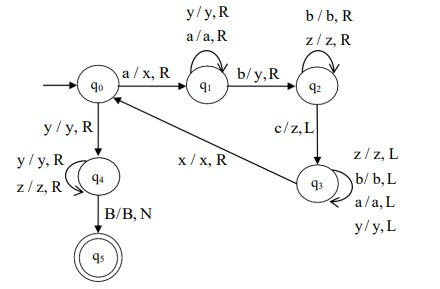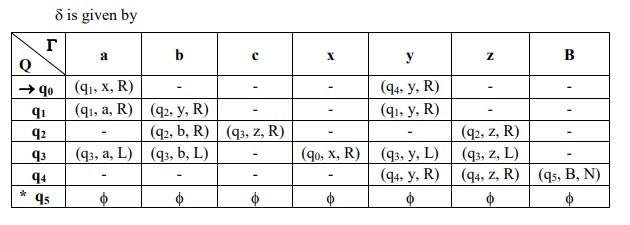# Construct a TM recognizing strings of the form an bn cn| n≥1 over = {a, b, c}

Data Structure AlgorithmsComputer ScienceComputers

## Algorithm

Step 1: Process the leftmost „a‟ and replace it by „x‟.

Step 2: Move right until the leftmost „b‟ is reached. Replace it by „y‟.

Step 3: Move right until the leftmost „c‟ is reached. Replace it by „z‟.

Step 4: Move left to reach the leftmost „a‟ and perform steps 1, 2 and 3 (n – 1) times.

Step 5: Halt if there are „n‟ number of x, y, z.

Turing Machine for the given language is as follows −The Turing machine, M is given by M = (Q, Σ, Γ, δ, q0, B, F)

Where,

• Q = {q0, q1, q2, q3, q4, q5}

• Σ = {a, b, c}

• Γ = {a, b, c, x, y, z, B}

• δ ⇒ Given by the transition diagram of the TM

• q0 = {q0}

• B = {B}

• F = {q5}